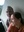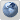Coffeehouse for desis
Would you like to react to this message? Create an account in a few clicks or log in to continue.

# The Map of Mathematics (my comments on FB)##The Map of Mathematics (my comments on FB)

...........................

(1) This mathematical map unfortunately does nor include the greatest discovery in math (0 or shunya) and its incorporation in the number system (as 9 +1 =10) giving rise to the decimal system (comprising just a small set of ten digits: 0, 1, 2, .., 9), leading the mankind on its path to progress in business, science and mathematics because of the ability of decimal system to uniquely define any number (no matter how large) in terms of only 10 digits (0-9) and also make possible and easy the mathematical operations (addition, subtraction, multiplication and division) using numbers.

(2) If there is going to be any map on mathematics, including the advances in math and interconnections in math branches (such as prime numbers and so on), then the mentioning of 0 and decimal system should be at the top, because without 0 and decimal system there wouldn't be anything (including the prime numbers and all the rest shown in the map).

(3) What's the significance of mentioning prime numbers on this map, which can't even be identified without the help of 0 based decimal system. Btw, the recent binary number system (using 0, 1) and hexadecimal number system (using 0, 1, 2....,9, A, B, C, D, E, F) also have their roots in ancient decimal system (using 0, 1, ..., 9). Why is it important to mention on the map the Riemann hypothesis (zeta function on prime number distribution), but not the decimal system (involving 0 or 10) without which the idea of prime numbers wouldn't even arise?

(4) There is no agenda here on who invented 0, but merely to show the extreme significance of 0 and the decimal system constructed through 0 (9 + 1 = 10) in making all the progress and advances in business, mathematics and science possible. Naturally, 0 and the decimal system (constructed through 0) need to find a place on the map of mathematics, especially if it explicitly mentions the prime numbers and Riemann hypothesis etc.Seva Lamberdar

Posts : 6533
Join date : 2012-11-29##Re: The Map of Mathematics (my comments on FB)Seva Lamberdar

Posts : 6533
Join date : 2012-11-29Permissions in this forum:
You cannot reply to topics in this forum